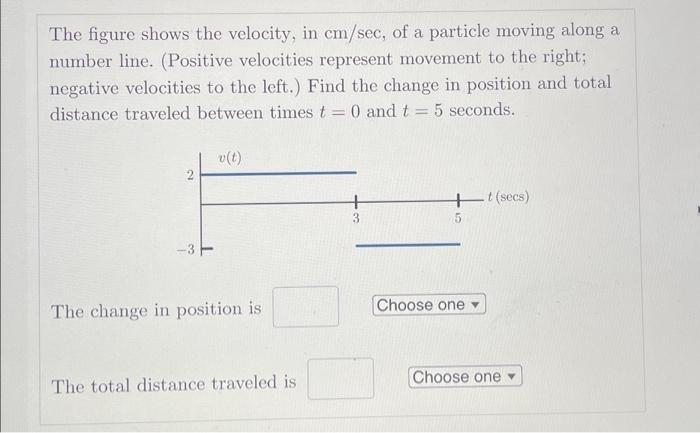Home / Expert Answers / Calculus / the-figure-shows-the-velocity-in-cm-sec-of-a-particle-moving-along-a-number-line-positive-veloci-pa127

# (Solved): The figure shows the velocity, in cm/sec, of a particle moving along a number line. (Positive veloci ...

The figure shows the velocity, in cm/sec, of a particle moving along a number line. (Positive velocities represent movement to the right; negative velocities to the left.) Find the change in position and total distance traveled between times t = 0 and t = 5 seconds. 2 -3 v(t) The change in position is The total distance traveled is 3 + t (secs) 5 Choose one Choose oneThe figure shows the velocity, in , of a particle moving along a number line. (Positive velocities represent movement to the right; negative velocities to the left.) Find the change in position and total distance traveled between times and seconds. The change in position is

We have an Answer from Expert